Mobile QR Code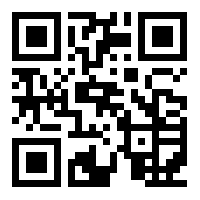1. (Department of Smart Robot Convergence and Application Engineering, Pukyong National University/ Busan, Korea hjkujm32@gmail.com )
2. (Department of Electronic Engineering, Pukyong National University/ Busan, Korea wlee@pknu.ac.kr )

Robot manipulator, Deep reinforcement learning, Optimal path, Robot simulator

## 1. Introduction

Reinforcement learning, supervised learning, and unsupervised learning are the three main approaches to machine learning. Deep reinforcement learning algorithms give machines the ability to generalize lessons learned during conventional reinforcement learning by expressing table-based value functions used in conventional algorithms through function approximation. This means that these systems can be applied to a variety of artificial-intelligence or large-scale engineering problems .

Deep reinforcement learning has been applied in the field of robotics when trying to perform elaborate and complex tasks. Currently, much research in this area is being conducted . However, since the robot's states and actions are inherently continuous, the dimensions of the problem space are very high, and as the number of dimensions increases, we encounter the curse of high dimensionality with exponentially increasing amounts of calculations and data.

As an example, consider a motor with 360 possible states. When there is only one motor, there are 360 states to deal with, but when there are n motors, there are $360^{n}$ states. Furthermore, if the motor has two actions (for example, it can rotate to either the left or right), the state-action space can become extremely large very quickly. These kinds of environments occur frequently in robotics, so for any deep reinforcement learning approach to derive optimal policies, it is necessary to reduce the dimensions of the state space or action-state space .

In this paper, we present a new method to search for an optimal path using real-time images as input to a deep reinforcement learning algorithm. The goal is controlling a robot manipulator to perform pick-and-place tasks. A pick-and-place operation refers to the process of picking up a certain object and putting it down at a desired location in the shortest possible time without colliding with any obstacles that may be in its path. In order to minimize the effect of high dimensionality, we assume that the robot is controlled through inverse kinematics using the position of its end effector. The position is obtained by analyzing images from a camera to define the robot’s state rather than using the angles given by the rotation of the motors on the robot manipulator.

We chose D3QN (Dueling Double Deep Q-Network (DQN)) as our deep reinforcement learning algorithm. The D3QN algorithm combines Double DQN and Dueling DQN, which offers performance improvements over conventional DQN [4,5]. Furthermore, by using PER (Prioritized Experience Replay), the efficiency of the experience replay memory was improved . This simplification of the work environment and the use of a powerful deep reinforcement learning algorithm enable a robot manipulator to perform pick-and-place tasks using an optimal path.

The rest of this paper is organized as follows. Section 2 and Section 3 describe the motivation and background of this work, respectively. In section 4, the simulation environment is described. Section 5 includes training results for the deep reinforcement learning algorithms used in this work and simulation results from the search for the optimal path of the robot manipulator’s end effector. Finally, Section 6 concludes the paper.

## 2. Motivation

DQN is the most representative deep reinforcement learning algorithm. It expresses the action value function through a deep neural network and has successfully learned to play computer games on an Atari 2600 using only high-dimensional raw images of the games as input. It has even achieved performance comparable to humans in some games .

There have been many attempts to use DQN for high-level robotic tasks, but so far, few have succeeded. This is due to the fact that most physical tasks take place in high-order action-state spaces with continuous action values . Nevertheless, there are some cases in which DQN along with some additional methods have been used experimentally to obtain good results when searching for optimal policies to provide to mobile robots and robot manipulators [9-11]. Therefore, we believe that an improved DQN algorithm and a simplified representation of the work environment would make it possible to find the optimal policy for pick-and-place operations by a robot manipulator.

## 3. Background

### 3.1 Markov Decision Process

The Markov Decision Process (MDP) is an ideal mathematical form that is able to elaborately express reinforcement learning problems theoretically . In MDPs, learners are called agents, and everything other than agents that interact with the learner is classed as the environment. The agent and the environment interact in every successive discrete time step. At every time step, the agent receives a representation of the state of the environment $s_{t}\in S$, where $S$ is the state space. The agent then selects an action $a_{t}\in A$ based on $s_{t}$, where $A~$ is the action space.

In the next time step the agent receives a reward function $r_{t+1}$ and the next state of the environment $s_{t+1}\in S$. At this time, the learner's goal is to maximize the gain $G_{t}$, which is the sum of the attenuated rewards:

##### (1)
$G_{t}=r_{t+1}+\gamma r_{t+2}+\gamma ^{2}r_{t+3}+\cdots =\sum _{k=0}^{\infty }\gamma ^{k}r_{t+k+1}$

where $\gamma \in \left[0,1\right]$ is the discount rate, which gives the present value of future compensation. The action value function evaluates the agent's action in a given state:

##### (2)
$q_{\pi }\left(s,a\right)=\mathrm{\mathbb{E}}_{\pi }\left[G_{t}|s_{t}=s,a_{t}=a\right]$

where $\pi$ is called the policy and the probability of choosing each possible action from that state. There is one policy that is better than or as good as all other policies, which is called the optimal policy $\pi _{*}$ and satisfies the following:

##### (3)
$q_{{\pi _{*}}}\left(s,a\right)=\max _{\pi }q_{\pi }\left(s,a\right)$

### 3.2 DQN

One of the main factors in the success of DQN is experience replay. First, the agent's experience $e_{t}\doteq \left(s_{t},a_{t},r_{t+1},s_{t+1}\right)$ is stored in the experience replay memory $D=\left\{e_{1},e_{2},\cdots ,e_{N}\right\}$ at every time step, where N gives the size of the experience replay memory. At a later point, during learning, experiences $e\sim D$ are uniformly randomly sampled from the memory, the number of experiences sampled is a predetermined parameter, and these experiences form a mini-batch that is entered as an input of the agent.

Due to experiential replay, the correlations between input data, which cause instability when reinforcement learning uses neural networks, are significantly reduced . A second factor in the success of DQN is the use of a separate target network. The target network has the same structure as the network in which learning happens but has independent $\theta ^{-}$ parameters, which are used to generate the target value $y$ used during learning:

##### (4)
$y=r+\gamma \max _{a'}q\left(s',a';{\theta ^{-}}_{i}\right)$

where $s'$ is the next state stored by $e=\left(s,a,r,s'\right)\sim D$, $a'$ is the action selected by the target network at $s'$, $i$ indicates how many times the update of the learning network parameters is repeated, and $\gamma$ is the discount rate. Due to the use of a target network, the parameters of the learning network in the i-th iteration are updated to minimize the loss function $L_{i}\left(\theta _{i}\right)$

##### (5)
$L_{i}\left(\theta _{i}\right)=\mathrm{\mathbb{E}}_{\left(s,a,r,s'\right)\sim D}\left[\left(y-q\left(s,a;\theta _{i}\right)\right)^{2}\right]$

### 3.3 Double DQN

Double DQN is a deep reinforcement learning algorithm that combines DQN with Double Q-learning. It is intended to reduce overestimation of Q-learning's action value function. This approach estimates the action value function more accurately and shows better performance than DQN in some Atari games .

This action value function overestimation is a problem caused by the maximization operation for the target value that is carried out while updating the current action value function. This problem causes significant deviations in the estimated action value function. Double DQN uses a target value $y$:

##### (6)
$y=r+\gamma q\left(s',\arg \max _{a'}q\left(s',a';\theta _{i}\right);{\theta ^{-}}_{i}\right)$

As shown in (6), the process of selecting an action and the process of evaluating the action are distributed to the learning and target networks to prevent overestimation of the action value function.

### 3.4 Dueling DQN

Dueling DQN uses a dueling architecture that has two streams for the state value function and advantage function. It combines these to estimate the action value function . The new action value function $q$ is then:

##### (7)
$q\left(s,a;\theta \right)=v\left(s;\theta \right)+\left(A\left(s,a;\theta \right)-\frac{1}{\left| A\right| }\sum _{a}A\left(s,a;\theta \right)\right)$

The dueling architecture has the following advantages. First, as the action value function is updated, the state value function is also updated. Therefore, other action value functions that have not been selected by the agent may also be updated frequently. Second, when updating the action value function, it is less affected by the difference in size between the target value and the current action value function, making the learning process more robust.

### 3.5 Prioritized Experience Replay

The prioritized experience replay method prioritizes and samples the data in the experience replay memory that will be used for learning according to the magnitude of the temporal difference error. This makes learning more efficient and effective compared to random uniform sampling . The priority of the samples to be stored in the experience replay memory is based on the magnitude of their temporal difference error (that is, how "surprising" the sample is). However, if only samples with high priority are greedily selected, and samples with low temporal difference errors are never selected, only a subset of the experience replay memory will ever be used. To solve this problem, stochastic sampling is used. The probability that the i-th sample in the memory is selected, $P\left(i\right)$, is given by:

##### (8)
$P\left(i\right)=~ \frac{{p_{i}}^{\alpha }}{\sum _{k}{p_{k}}^{\alpha }}$

where $p_{i}$ is the priority of the i-th sample, and the constant $\alpha ~$determines how much effect priority has on a sample’s chance of being selected.

## 4. Optimal Path Search Method

### 4.1 Simulation Environment

The robot manipulator and environment used in our simulation were created using the realistic robot simulator Webots . Webots is a professional robot simulation software package that provides a fast prototyping environment that allows users to create 3D virtual worlds by defining physical properties such as the mass, type of joint, coefficient of friction, and so on. In addition, various sensors and actuator devices such as distance sensors, drive wheels, cameras, motors, and touch sensors can be modeled. Webots is a more realistic simulator than other simulation tools available. Webots not only allows you to simulate the physical properties of the environment, but also provides realistic photo-like camera images that reduce the gap between the real world and the simulated environment. Due to these advantages, Webots is a great simulation tool for reinforcement learning researchers. In addition, a useful framework for developers called Deepbots has also been created for this software .

Fig. 1 shows the robot manipulator, which is a three-axis robot that has two linear motors to operate the gripper. In the work environment, both objects to be moved and obstacles are on a table. Our goal is to bring the target object to the target point while avoiding obstacles in the shortest time. A camera to monitor the work environment hangs from the ceiling.

### 4.2 Proposed Method

The camera in Webots supports object detection, recognizes target objects, and allows us to track their positions using images. Based on information obtained from images captured by the camera, a new representation is created, as shown in Fig. 2. Fig. 2 shows the position of the target object, the position of the obstacle, and the destination expressed as a grid world composed of square boxes. The grid world has dimensions of 12x12 and is created as an 84x84 image. This grid world image becomes the input image to the training network of the deep reinforcement learning algorithm, which will search for the optimal path to complete the pick and place tasks.

As shown in Fig. 3, the robot simulator extracts object information from the input image of the camera. Then, a new grid world image is created and delivered to the deep reinforcement learning agent. After receiving the image, the agent selects the next action and transmits it to the robot simulator. The robot simulator calculates the position where the end effector should move based on that action and then moves each joint through inverse kinematics. Next, a new image is then recorded by the camera after the robot has moved, and the information obtained from it is used to create the new grid world image. This process repeats from the moment the object is picked up to the moment it is put down on the target point.

In reinforcement learning, there are various episodes, and learning takes place from the experiences that are created within them. It takes a considerable amount of time to acquire sufficient experience for a robot to learn a skill well while in the simulation tool. To tackle this problem, we created training data using OpenAI Gym frame, a toolkit for developing and comparing reinforcement learning algorithms . OpenAI Gym frame allows you to test your own reinforcement learning algorithms using various internal game environments. Furthermore, if you use OpenAI Gym frame, you can create your own game environment where your algorithms can learn how to complete tasks. Since we represented the work environment using a grid world image, we were able to create virtual training data and significantly cut the time required for training.

## 5. Simulation Results

### 5.1 Training of Deep Reinforcement Learning Algorithms

We let the deep reinforcement learning algorithm learn two environments. In the first environment, the starting point and destination are randomly generated, and there are no obstacles in the way. The scalar reward function for this is as follows.

##### (9)
r t + 1 = 0, r e a c h    d e s t i n a t i o n 0 . 1, o t h e r w i s e

The robot has a maximum of 500 time steps per episode, and the worst total reward per episode is -50. The goal of the robot is to reach the destination in the shortest time. The training was conducted over a total of 50,000 episodes, where two deep reinforcement learning algorithms were trained: DQN and D3QN with PER. The results achieved by these two algorithms in this task are shown in Fig. 4.

The vertical axis represents the total reward per episode, and the horizontal axis represents the number of episodes. For each episode, the total reward from the most recent 1000 episodes was averaged and used as the output. In a simple environment with no obstacles, both algorithms successfully learned how to compete the task well. By episode 50,000, the average reward was about -0.65, which means that the destination was reached in 6 to 7 time steps on average. The overall average and highest average rewards of D3QN with PER were slightly higher than those of DQN.

Table 1. Simulation hyperparameters.

 Hyperparameters Values Architecture Conv(32-8x8-4) Conv(64-4x4-3) Conv(64-3x3-1) Fully Connected(512) Fully Connected(256) Fully Connected(64) Batch size 128 Start $\epsilon$ 1.0 End $\epsilon$ 0.1 Annealing step 500,000 Memory size 500,000 Learning rate 0.0001 Discount rate 0.99 PER $\alpha$ 0.6 PER $\beta$ 0.4 PER $\beta$ increasement 0.0000025

The second environment is a more complex and difficult environment than the first one. In the second environment, three obstacles appear stochastically with random positions and sizes. The goal of the robot is to pick up the target and move it to the destination in the shortest time possible (measured in steps) while avoiding obstacles. If the robot collides with an obstacle, it returns to its original position. This is more difficult than the first environment because the agent needs to know where the obstacles are in the work environment and to find a path to avoid them. The reward function and the learning process are the same as in the first environment. The results achieved by the two algorithms in this task are shown in Fig. 5.

Fig. 5. Training results for complex environment: (a) Comparison of training results of DQN and D3QN with PER during 10,000 episodes; (b) Training result of D3QN with PER during 50,000 episodes.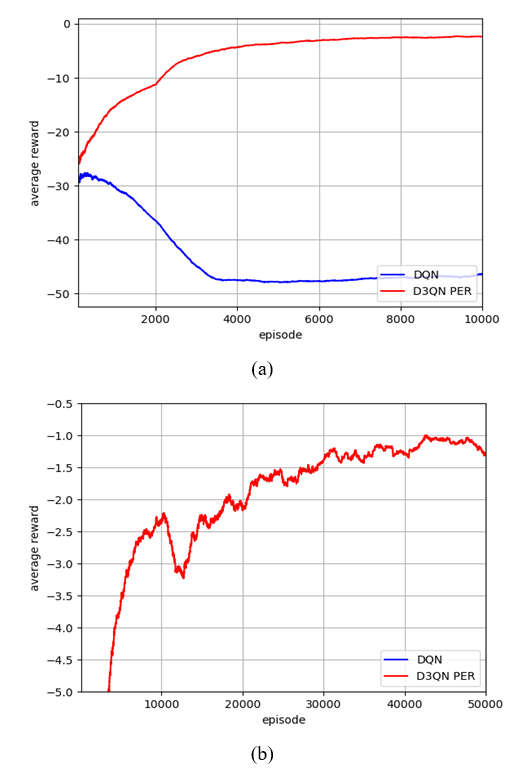Fig. 6. Optimal path when there is no obstacle in the workspace.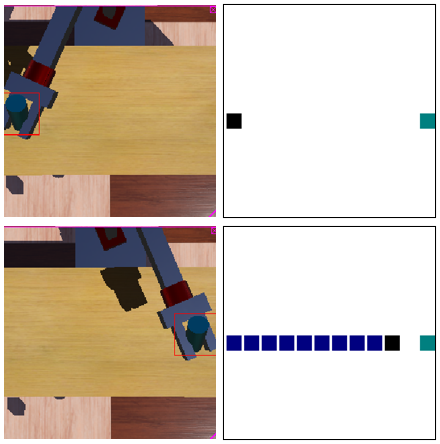In Fig. 5, the difference between the two algorithms can be seen clearly. As the learning progressed, DQN was not able to find the destination and could only avoid obstacles. Eventually, we decided that DQN would not be able to complete the task and ended training in the 20,000th episode. In contrast, D3QN with PER successfully learned how to complete the task. It can be seen that the average reward is about -1.2 by the 50,000th episode, which indicates that the robot was able to reach the destination by moving 12 times on average. To teach the 3-axis robot manipulator, the weights found by the deep reinforcement learning algorithm model in Gym Frame were used. Table 1 shows the hyperparameters for Double Dueling DQN with PER used for training.

### 5.2 Optimal Path Search

Figs. 6-8 show the optimal path search results of the 3-axis robot manipulator that was taught using the stored weights of the trained model. These were passed to the deep reinforcement learning algorithm model, which was then able to interact with the working environment implemented in the simulation. The first test was to ensure that the optimal path could be found when there were no obstacles. Fig. 6 shows that the robot manipulator is able to find the fastest straight path when it picks up and moves the object to the target.

Fig. 7. Optimal path when there are static obstacles in the workspace.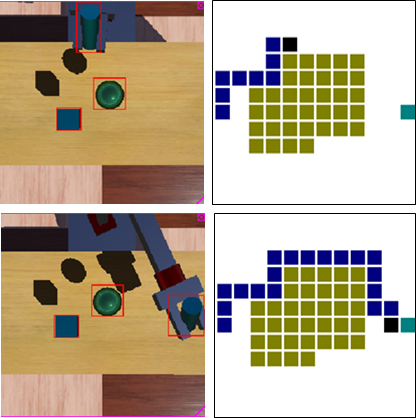Fig. 8. Optimal path when there are dynamic obstacles in the workspace.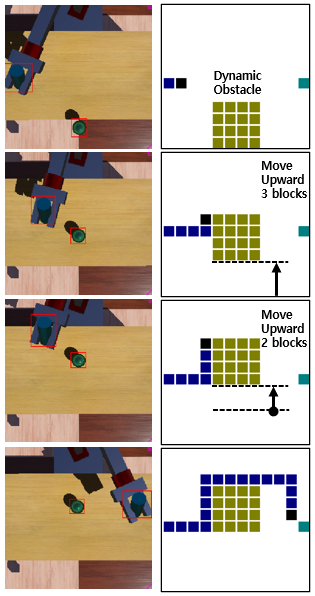The second test was to check that our model could find the optimal path when there were static obstacles. There are two obstacles in the work environment. The tests confirmed that the robot manipulator is able to avoid obstacles and reach the destination. In Fig. 7, the robot follows the fastest path while avoiding collisions with the obstacles.

The final test was to ensure that our model could find the optimal path when there were moving obstacles. One obstacle moves and blocks the simple path to the target while the robot manipulator performs a pick and place operation. As shown in Fig. 8, the robot manipulator moved along the path with the greatest reward while making corrections to the optimal path in real time to avoid the moving obstacle.

## 6. Conclusion

In this paper, we proposed a method that searches for the optimal trajectory for a robot manipulator to take in pick-and-place tasks using deep reinforcement learning with images of the workspace as inputs. This method was successful even when there were moving obstacles in the workspace. We confirmed that the deep reinforcement learning agent is able to find optimal behavior using a simulation.

It was also shown that optimal robot manipulator operation can be achieved using a variant of DQN. This kind of robot manipulator operation is considered difficult when using DQN, but when applying improved DQN algorithms, such as Double DQN and Dueling DQN, the task becomes more feasible. The method proposed in this paper is expected to be applicable to pick-and-place tasks and a wide variety of applications using robot manipulators.

### References

1
Sutton Richard S., Andrew G. Barto., 2018, Reinforcement learning: An introduction., MIT press2
Zhao Wenshuai, Jorge Peña Queralta, Tomi Westerlund., 2020, Sim-to-Real Transfer in Deep Reinforcement Learning for Robotics: a Survey., 2020 IEEE Symposium Series on Computational Intelligence (SSCI). IEEE3
Kober Jens, Bagnell J. Andrew, Peters Jan, 2013, Reinforcement learning in robotics: A survey., The International Journal of Robotics Research, Vol. 32, No. 114
Hasselt Hado van, Guez Arthur, Silver David, 2016, Deep reinforcement learning with double q-learning., Proceedings of the AAAI Conference on Artificial Intelligence., Vol. 30, No. 15
Wang Ziyu, et al. , 2016, Dueling network architectures for deep reinforcement learning., International conference on machine learning. PMLR6
Schaul Tom, et al. , 2015, Prioritized experience replay., arXiv preprint arXiv:1511.05952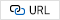7
Mnih Volodymyr, et al. , 2015, Human-level control through deep reinforcement learning., nature 518.7540, pp. 529-5338
Gu S., Holly E., Lillicrap T., Levine S., 2017, Deep reinforcement learning for robotic manipulation with asynchronous off-policy updates, 2017 IEEE International Conference on Robotics and Automation (ICRA) Singapore, pp. 3389-33969
Zhang F., Leitner J., Milford M., Upcroft B., Corke P., 2015, Towards Vision-Based Deep Reinforcement Learning for Robotic Motion Control., ArXiv abs/1511.03791.10
James S., Johns E., 2016, 3D Simulation for Robot Arm Control with Deep Q-Learning., ArXiv abs/1609.03759.11
Xin J., Zhao H., Liu D., Li M., 2017, Application of deep reinforcement learning in mobile robot path planning, 2017 Chinese Automation Congress (CAC) Jinan China, pp. 7112-711613
Kirtas M., et al. , 2020, Deepbots: A Webots-Based Deep Reinforcement Learning Framework for Robotics., IFIP International Conference on Artificial Intelligence Applications and Innovations. Springer Cham## Author

Yungmin Sunwoo received his B.S. degree in Electronic Engineering from Pukyong National University. Cu-rrently, he is a graduate student in Smart Robot Convergence and Application Engineering at Pukyong National University.

Won Chang Lee received B.S. in Control and Instrumentation Engi-neering from Seoul National University in 1983. He received and M.S. in Electrical and Electronic Engineering from Korea Advanced Institute of Science and Technology (KAIST) in 1985, and Ph.D. in Electrical Engineering from Pohang University of Science and Technology(POSTECH) in 1992. He was with Korea Research Institute of Standards and Science from 1985 to 1988. He is currently a professor in the Department of Electronic Engineering at Pukyong National University, Busan, South Korea. His current areas include nonlinear control, robotic systems, and artificial intelligence.